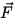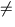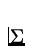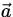# Newton's Second Law

If0 (i.e. there is a net external force acting on an object) then,= m. (2)
Definition: Inertia is the tendancy of an object to resist any attempt to change its state of motion. Mass is the force required per unit of acceleration produced and is a measure of inertia. Mass is a scalar and has SI units of kilograms (kg). Example: If a bowling ball and a golf ball are hit with a bat, the bowling ball would be much harder to get moving since it has greater mass and thus greater inertia.

Note:

•is inversely proportional to m . This means that, for the same force, a smaller mass will have a larger acceleration.
• Newton's second law is a vector equation which contains three scalar equations (in three dimensions):Fx = max ,Fy = may ,Fz = maz .
• The first law is a special case of the second law.
• The SI unit of force is the Newton (N). Definition: 1 Newton is the force that produces an acceleration of 1 m/s 2 when acting on a 1 kg mass. In the cgs system: 1 dyne = 1 g cm/ s 2= 10- 5N. In the British engineering system: 1 pound (lb) = 4.448 N.

Definition: Weight () is the force exerted on an object by a gravitational field. From Newton's second law,

 w = mg. (3)
Note:
• Weight is a vector with direction towards the earth's center, or perpendicular to the earth's surface.
• The weight of an object is different on the earth and on the moon since the strength of the gravitational field is different ( gearthgmoon ).
• The value of g varies with distance from the center of the earth (more on this in chapter 7). As a consequence:
• Since the earth isn't a perfect sphere, the weight of an object varies slightly from place to place on the earth's surface.
• The weight of an object varies slightly with altitude above the earth's surface.
• In comparison, mass is a scalar with a value independent of location. Notice however that, in the approximation that g is constant, mass is proportional to the magnitude of the weight and the two quantities can be used interchangeably. This is called the equivalence principle.Next: Newton's Third Law Up: Force Previous: Newton's First Law